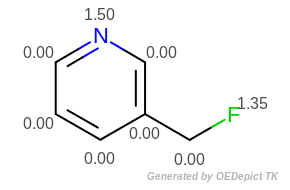# Periodic Table Functions¶

To simplify the task of dealing with the elements of the periodic table, OEChem TK contains several functions to obtain useful properties of the elements.

## Atomic Symbol/Atomic Number¶

A common task is to obtain or display the atomic symbol of an atom represented by an `OEAtomBase`. To save space and reduce redundancy and consistency issues, the `OEAtomBase` class contains only an ```unsigned integer``` representing the atom’s atomic number. This value may be obtained using the `OEAtomBase.GetAtomicNum` method. This value can be converted into an atomic symbol using the `OEGetAtomicSymbol` function.

```String symbol = oechem.OEGetAtomicSymbol(OEElemNo.C);
System.out.println("The atomic symbol for carbon is " + symbol);
```

The example above uses the integer constant `OEElemNo.C` from the `OEElemNo` namespace. This namespace represents the atomic numbers of the 111 elements as their symbols as a convenience.

The inverse of `OEGetAtomicSymbol`, i.e. obtaining the atomic numbers from an atomic symbol, is performed by the `OEGetAtomicNum` function.

```System.out.println("The atomic number for 'Cl' is " + oechem.OEGetAtomicNum("Cl"));
```

## Element Properties¶

OEChem TK provides several functions for obtaining properties of the elements, all of which take an unsigned integer argument representing the element’s atomic number.

Property Name

Return Value

Function

Average Atomic Weight

double

`OEGetAverageWeight`

Most Abundant Isotope

unsigned int

`OEGetDefaultMass`

The OEChem TK library also provides the following two functions to deal with specific isotopes:

Both functions take an unsigned integer representing the isotope’s atomic number (number of protons), and an unsigned integer representing the isotope’s mass (number of protons plus neutrons).

The following example shows how to calculate molecular weight (`OECalculateMolecularWeight`) :

```static double CalculateMolecularWeight(OEMolBase mol) {
int elemno = 0;
int mass = 0;
int implicitH = 0;
double weight = 0.0;

for (OEAtomBase atom : mol.GetAtoms()) {
elemno = atom.GetAtomicNum();
mass = atom.GetIsotope();
implicitH += atom.GetImplicitHCount();
if ((elemno != 0) && (mass != 0) && oechem.OEIsCommonIsotope(elemno, mass)) {
weight += oechem.OEGetIsotopicWeight(elemno, mass);
} else {
weight += oechem.OEGetAverageWeight(elemno);
}
}
weight += implicitH * oechem.OEGetAverageWeight(OEElemNo.H);
return weight;
}
```

Property Name

Get Method

Applied on `OEMolBase`

Reference

`OEGetCovalentRadius`

`OEAssignCovalentRadii`

`OEGetDelphiRadius`

`OEAssignDelphiRadii`

Accelrys

`OEGetHonigIonicCavityRadius`

`OEAssignHonigIonicCavityRadii`

[Rashin-1985]

`OEGetBondiVdWRadius`

`OEAssignBondiVdWRadii`

[Bondi-1964]

`OEGetPaulingVdWRadius`

`OEAssignPaulingVdWRadii`

[Pauling-1960]

N/A 

`OEAssignZap9Radii`

[Nicholls-2008]

`OEAssignZap7Radii`

[Nicholls-2010]

Generic Assignment Function

N/A 

`OEAssignRadii`

Table footnote:

 The get method is not available, since the radius value of `OEElemNo.O` and `OEElemNo.N` (defined in [Nicholls-2008]) depend on the chemical environment of the given atom.

 Can be used to call any of the other methods to assign radii as well as to clear atomic radii.

The following snippet shows how to set and access the “Pauling” van der Waals radius in a given `OEMolBase`:

```oechem.OEAssignPaulingVdWRadii(mol);
for (OEAtomBase atom : mol.GetAtoms()) {
System.out.println(atom.GetIdx() + " " + atom.GetRadius());
}
```Example of setting “Pauling” van der Waals radius